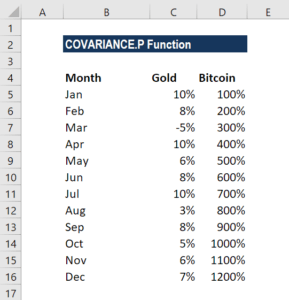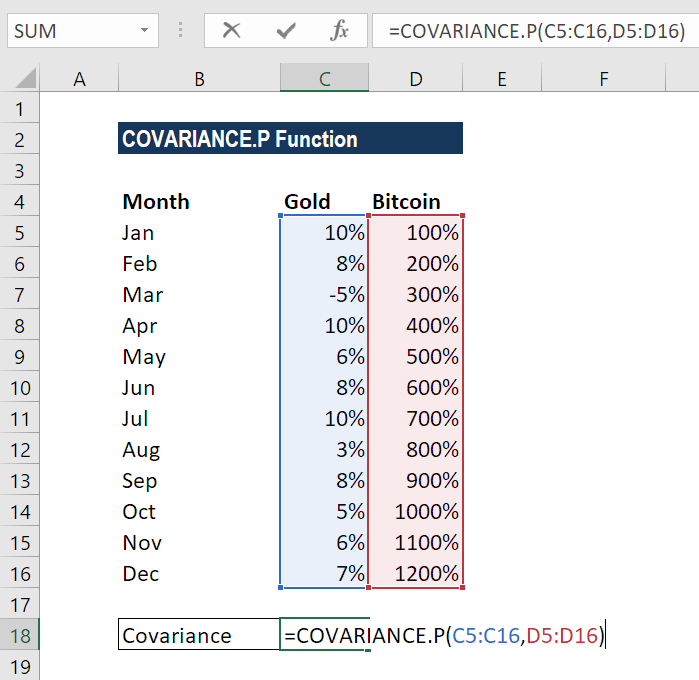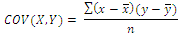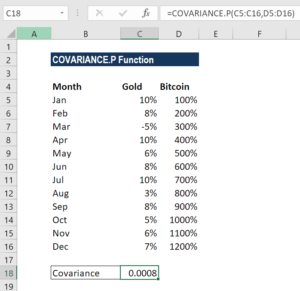# COVARIANCE.P Function

Calculates the covariance of two given set of values

## What is the Covariance Excel Function?

The covariance Excel function is categorized under Statistical functions. It calculates the joint variability of two random variables, given two sets of data.

Suppose, as a financial analyst, that we wish to determine whether greater income accompanies greater levels of education in a population or not. In such a scenario, we can use the COVARIANCE.P function. It was introduced in MS Excel 2010 to replace COVAR, with improved accuracy over its predecessor.

### Covariance Formula in Excel

=COVARIANCE.P(array1, array2)

The COVARIANCE.P function uses the following arguments:

1. Array1 (required argument) – This is a range or array of integer values.
2. Array2 (required argument) – This is a second range or array of integer values.

A few things to remember about the arguments:

• If the given arrays contain text or logical values, they are ignored by the COVARIANCE in Excel function.
• The data should contain numbers, names, arrays, or references that are numeric. If some cells do not contain numeric data, they are ignored.
• The data sets should be of the same size, with the same number of data points.
• The data sets should not be empty, nor should the standard deviation of their values equal zero.

### How to use the Covariance Excel Function

To understand the uses of the function, let us consider a few examples:

#### Example 1 – Covariance Excel

Suppose we are given the monthly returns of two assets, gold and bitcoin, as shown below:We wish to find out covariance in Excel, that is, to determine if there is any relation between the two. The relationship between the values in columns C and D can be calculated using the formula =COVARIANCE.P(C5:C16,D5:D16).Covariance in Excel is a statistical measurement of the strength of the correlation between two sets of variables, and is calculated by the following equation:Where:

• and y are the sample means (averages) of the two sets of values
• is the sample size

The formula gives the result 0.0008, which indicates a negative correlation between the two assets.### Notes about the Covariance Excel Function

1. The function is available from MS Excel 2010. However, it is simply an updated version of the COVAR function that is available in earlier versions of Excel.
2. #VALUE! error – Occurs when one or both of the supplied data arrays are empty.
3. #N/A error – Occurs if the given arrays are of different lengths.

Thanks for reading CFI’s guide to important Excel functions! By taking the time to learn and master these functions, you’ll significantly speed up your financial analysis. To learn more, check out these additional CFI resources:

• Excel Functions for Finance